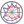Course title
Differential and Integral Calculus 1
 yamazawa hiroshihirose sampeinakagawa takahirohoriuchi tomohiroCourse description
In this class, students learn the basic concepts and operations of one-variable functions. The first part deals with limits, derivatives, and extrema, and the second part deals with indefinite and definite integrals for various functions. Students also learn the basic properties of important functions such as polynomials, exponential functions, logarithmic functions, trigonometric functions, and inverse trigonometric functions.
Note that these also appear in the fundamentals of statistics and even data science.
Purpose of class
The purpose of this class is to understand the meaning of calculus of one-variable functions, and to be able to calculate them.
Goals and objectives
1. To be able to calculate limit
2. To be able to understand the meaning of differentials and related operations, and calculate them.
3. To be able to calculate differential, derivative test, and extrema of functions related to statistics.
4. To be able to understand the meaning of indefinite and definite integrals, and calculate them.
5. To be able to calculate indefinite and definite integrals related to statistics.
Language
Japanese
Class schedule

Class schedule HW assignments (Including preparation and review of the class.) Amount of Time Required
1. Limit and continuity of elementary functions such as polynomials Read through the textbook p.23-p.30 before class. Solve exercises after class. 190minutes
2. Basic properties of differential Read through the textbook p.33-p.42 before class. Solve exercises after class. 190minutes
3. Trigonometric function Read through the textbook p.45-p.50 before class. Solve exercises after class. 190minutes
4. Inverse trigonometric function Read through the textbook p.53-p.62 before class. Solve exercises after class. 190minutes
5. Exponential function, logarithmic function, and their relationship to statistics Read through the textbook p.65-p.71 before class. Solve exercises after class. 190minutes
6. Derivative test and extremum Read through the textbook p.84-p.91, p.93-p.99 before class. Solve exercises after class. 190minutes
7. Midterm examination and its explanation Preparation of midterm examination 190minutes
8. Indefinite integral of elementary functions such as polynomials Read through the textbook p.113-p.118 before class. Solve exercises after class. 190minutes
9. Indefinite integration by substitution and by parts Read through the textbook p.121-p.126 before class. Solve exercises after class. 190minutes
10. Indefinite integral of trigonometric functions Read through the textbook p.128-p.132 before class. Solve exercises after class. 190minutes
11. Indefinite integration of rational and irrational functions Read through the textbook p.134-p.142 before class. Solve exercises after class. 190minutes
12. Definitive integral and its use (mean) Read through the textbook p.146-p.152 before class. Solve exercises after class. 190minutes
13. Calculation of definite integral Read through the textbook p.153-p.161 before class. Solve exercises after class. 190minutes
14. Final examination and their explanations Preparation of final examination 190minutes
Total. - - 2660minutes
Relationship between 'Goals and Objectives' and 'Course Outcomes'

Midterm examination Final examination Reports and little examinations Total.
1. 6% 3% 9%
2. 29% 12% 41%
3. 0%
4. 35% 15% 50%
Total. 35% 35% 30% -
Evaluation method and criteria
Midterm examination(35%), Final examination(35%), Reports and little examinations(30%)
Textbooks and reference materials
Textbook: 微分積分改訂版、矢野健太郎、石原繁編、裳華房
Prerequisites
Review high school mathematical contents
Office hours and How to contact professors for questions
• 30 minutes after class
Regionally-oriented
Non-regionally-oriented course
Development of social and professional independence
• Course that cultivates an ability for utilizing knowledge
Active-learning course
More than one class is interactive
Course by professor with work experience
Work experience Work experience and relevance to the course content if applicatable
N/A 該当しない
Education related SDGs:the Sustainable Development Goals• 4.QUALITY EDUCATION